# Distracted Driver Detection

Source: Deep Learning on Medium

### I. Definition

#### I.I. Project Overview

One efficient way to reduce car accidents is preventing distracted driving, which is the act of driving while engaging in other activities such as texting, talking on the phone, etc. Activities of that nature distract the driver from paying attention to the road. These distractions in turn compromise the safety of the driver, passengers, bystanders and others in other vehicles. The United States Department of Transportation states that one in five car accidents are caused by distracted drivers, meaning that distracted driving causes injuries to 425,000 people and claims the lives of 3000 others every year¹.

In this project, a set of machine learning models were developed and refined for the purpose of detecting drivers’ activities while driving and classify them, by training them on a dataset obtained from an insurance company called State Farm.

#### I.II. Problem Statement

In order to improve the alarming statistics states in the project overview section, innovative methods should be tested. One such method would be to develop an algorithm to detect drivers engaging in distracted behaviours by feeding it 2D dashboard camera images. This algorithm can then be used as an API in a device to classify the driver’s behaviour by checking if they are driving attentively, wearing their seatbelt and remind them if they are not.

The dataset provided by State Farm consists of images, which means that the most efficient way to tackle the problem at hand is to develop a deep convolutional neural network model and then train it on a training subset of the dataset with the objective to optimize a certain evaluation metric. The model will then be tested on a testing subset of the dataset and evaluated.

#### I.III. Metrics

The models will be evaluated using the multi-class logarithmic loss between the predicted class of the image and the actual class. The formula of the evaluation metric is:

Where N is the number of images in the dataset, M is the number of image class labels, log is the natural logarithm, y is 1 if observation i belongs to class j and 0 otherwise, and p is the predicted probability that observation i belongs to class j.

The logarithmic loss measures the performance of a classification model by taking the prediction input as a probability value between 0 and 1 rather than a simple true or false. That means the logarithmic loss takes into account the uncertainty of the model prediction based on how much it varies from the actual label, yielding a more nuanced evaluation of the model’s performance.

### II. Analysis

#### II.I. Data Exploration

The dataset used in this project was provided by State Farm through a Kaggle competition, which if a set of images of drivers taken inside a car capturing their activities such as texting, talking on the phone, eating, reaching behind, putting on makeup, etc. These activities are classified into 10 classes as:

• + c0: safe driving
• c1: texting — right
• c2: talking on the phone — right
• c3: texting — left
• c4: talking on the phone — left
• c5: operating the radio
• c6: drinking
• c7: reaching behind
• c8: hair and makeup
• c9: talking to a passenger

The dataset contains a total of 102150 images split into a training set of 22424 images and a testing set of 79726 images. Here is a sample of the dataset images.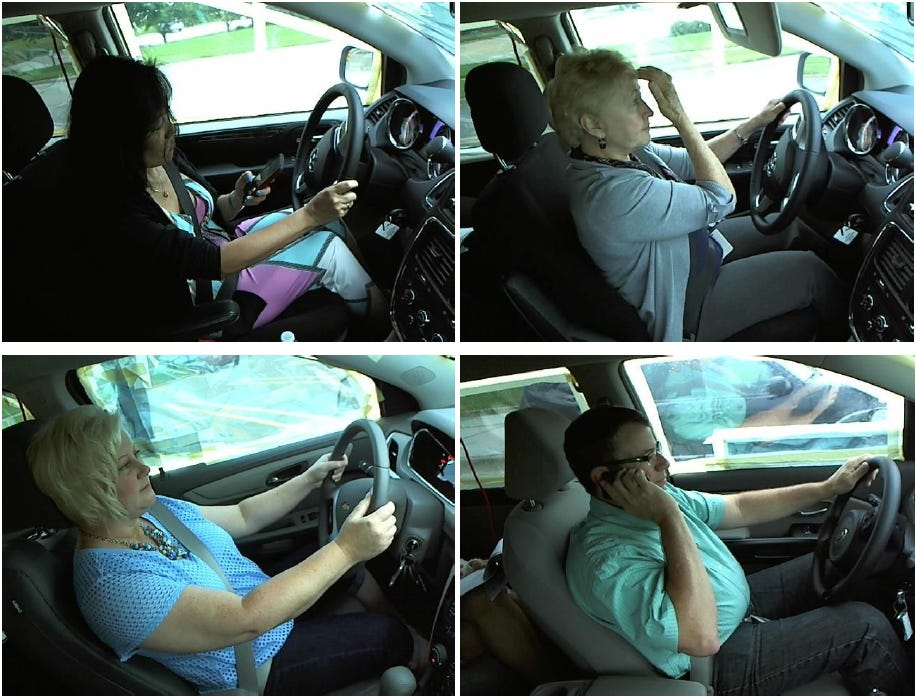Figure 1. A sample of the dataset.

The images are 480 X 640 pixels and the distribution of the classes in the training set are relatively uniform. The total size of the dataset is 4 GB, which is publicly available at:

The data contains three files:

• + imgs.zip — zipped folder of all (train/test)
• images sample_submission.csv — a sample submission file in the correct format for Kaggle submission.
• driver_imgs_list.csv — a list of training images, their subject (driver) id, and class id

#### II.II. Exploratory Visualization

One important factor in choosing an evaluation metric and a validation method is the uniformity of the target class distribution. The class distribution for this dataset is relatively uniform as can be seen from figure 2. This allows us to use one model for training the whole dataset, as if it was not uniform a separate binary classification model would have been used for each class individually then the evaluations of these models would be averaged to get a final evaluation of the classification approach. Moreover, the data being uniform makes drawing a validation set a simple task such that splitting a certain percentage from the shuffled training set would yield a set of similar characteristics.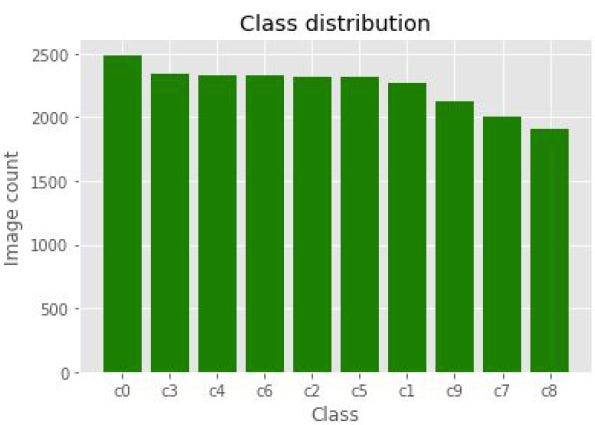Figure 2. Class distribution of the data set.

#### II.III. Algorithms and Techniques

The project was executed using a convolutional neural network (CNN). CNNs or ConvNets are made of neurons that have learnable weight and biases, each neuron receives an input, performs a dot product and usually followed by a non-linear function. The inputs of ConvNets are image pixels as vectors and its outputs are class scores. As the vectors go from the input layer to the output layer they pass through a series of hidden layers, each hidden layer consists of neurons where each neuron is fully connected to every neuron in the previous layer while remaining completely independent from the other neurons in the same hidden layer.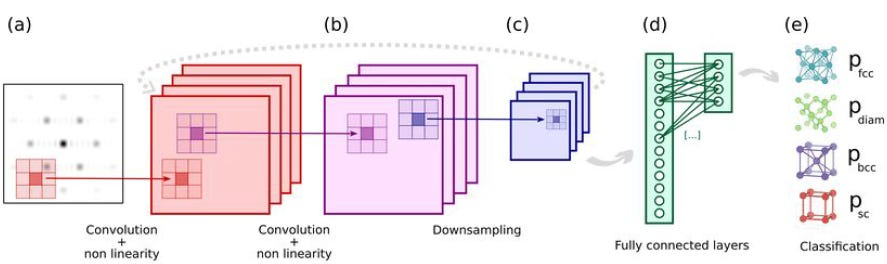Figure 3. Visual representation of CNN.

CNN differs from other neural networks by arranging its neurons in three dimensions (height, width, depth). The main layer types used in CNN are:

1. Convolutional layer: computes the output of a neuron that is connected to the region in the input by applying a dot product between its weights and the region’s values.
2. Pooling layer: performs a downsampling operation along the spatial dimensions (i.e. height and width).
3. Fully connected layer: computes the class score.

A popular method in computer vision tasks called transfer learning was also used to tackle the problem stated in this project. Transfer learning is the process of storing knowledge gained while solving one problem and applying it to a different but similar problem. There are two major approaches to transfer learning:

1. Using pre-trained CNN as fixed feature extractor: This technique uses a CNN pre-trained on a large dataset such as Imagenet, drops the last fully connected layer then uses the rest of network as feature extractor for a different dataset. For every image in the new data, this process would compute a vector of certain dimension depending on the pre-trained model architecture, the computed vector would contain the activation weights of the hidden layers just before the classifier, which are called CNN codes. After extracting the CNN codes a linear classifier would be trained for the new dataset.
2. Fine-tuning a pre-trained CNN: This approach differs from the previous one by fine-tuning the weights of pre-trained network by continuing the backpropagation, which can be done either by fine-tuning the whole network or freezing the earlier layers and only fine tune the higher level layers of the network, the latter serves two purposes one of them is that by keeping the first layers fixed it regularizes the model preventing it from overfitting the data, the other is that reduces the training time as the earlier layers contains generic features such as edges and curves which is applicable to most application.

#### II.IV. Benchmark

The benchmark model for this project was a simple CNN model made up of three blocks each block starts with a convolutional layer applying a relu activation followed by a max-pooling layer. The data is then flattened using a flatten layer followed by a dropout layer followed by a dense layer followed by another dropout layer and finally another dense layer of 10 outputs corresponding to the number of classes and a softmax activation. The model was compiled using a rmsprop optimizer and a categorical cross-entropy loss function. The model was trained for 4 epochs with batch size 32. The model performed well achieving a logarithmic loss of 0.04 on the validation dataset and a logarithmic loss of 0.2 on the test set.

### III. Methodology

#### III.I. Data Preprocessing

Initially, the project was intended to use the whole dataset but during preprocessing the data, the virtual instance has run out of memory as just preprocessing the training set which holds 22424 images has taken over 20 GB of memory. The instance has a memory of 61 GB and the test set holds just under 80000 images with no labels, meaning that to test the model on the test set its predictions had to be submitted to Kaggle. Therefore, a subset of the test dataset cannot be used as this would result in incomplete Kaggle submission. This problem was resolved by considering the training dataset as the entire dataset then splitting 10% of it as a validation set and another 10% as a testing set. Moreover, the project was set to use keras with tensorflow as backend which means that the input has to be a 4D tensors to be compatible with keras’ CNN, so the images were first resized to 224 x 224 pixels, then converted into a 3D tensors and then into a 4D tensors of shape (N, 224, 224, 3) where N is the number of images. After that, the tensors were scaled by dividing them over 255.

#### III.II. Implementation

The benchmark model was implemented first by creating a model through the use of keras’ function Sequential and adding a Convolutional layer of 16 filters, kernel size 2X2, same padding, relu activation and input shape the same as the 4D tensors that are (None, 224, 224, 3). A max-pooling layer with pool size 2X2 was added after that a couple more Convolutional layer were also added each followed by a 2X2 max-pooling layer. The difference between the first Conv layer and the last two is that no input shape was specified as that only required for the first layer of the model, and the number of filters was double the previous layer as shown in figure 4. The model was regularized by adding Dropout layer of rate 0.3 followed by a Flatten layer then a Dense layer of 80 neurons with a relu activation. The output was regularized by another Dropout layer of 0.4 rate and finished with a Dense layer of 10 outputs representing the data classes with a softmax activation.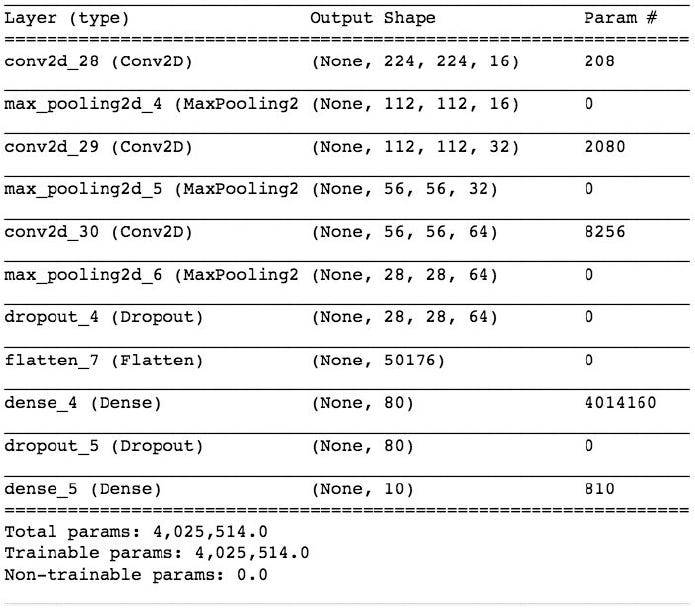Figure 4. The summary of the benchmark model.

The model was compiled using a rmsprop optimizer and categorical cross-entropy loss function then was trained on the training data and validated on the validation data for 4 epochs of batch size 32. During training, the weights that achieved the best log loss score on the validation data were saved in an hdf5 file. These were loaded into the model to test them on the test set after importing a log loss function from sklearn’s metrics. The predictions of the model were then compared to the actual labels in terms of logarithmic loss.

The initial solution started by importing a pre-trained CNN model. The chosen model was Xception as it is the second best performing model in terms of accuracy with less than half the parameters of the best model. The Xception model was trained on Imagenet†a massive dataset with more than 1.2 million images of 1000 categories. The last layer was removed as it was the classifier of the 1000 categories. The CNN codes of all the images in the three datasets were then extracted by using keras’ predict function after running the tensors through preprocess_input another keras function. A second model was created using keras’ Sequential function where a Dropout layer with rate 0.3 and input shape matching the CNN codes shape that is (None, 7, 7, 2048) was added to the model followed by a Flatten layer and Dense layer with 10 outputs corresponding to the number of the dataset classes and a softmax function. The model was compiled using a rmsprop optimizer and categorical cross-entropy loss function then was trained on the training data and validated on the validation data for 20 epochs of batch size 16 while in the process saving the best performing weights on the validation set. The testing was carried out in the same manner as in the benchmark model.

#### III.III. Refinement

The first attempt to refine the results was to keep using the pre-trained Xception model as a feature extractor but train the CNN codes further before classification. To do so a new model was created using Sequential function where a Dropout layer of 0.3 rate and input shape of the same dimensions as the CNN codes were added. Then the data was flattened by adding a Flatten layer to the model followed by a Dense layer of 250 outputs and a relu activation, to regularize the output of the dense layer another Dropout layer of 0.4 was added to the model. The model was finished by a classifying layer of 10 outputs and a softmax activation. The model was compiled with an Adam optimizer and categorical cross-entropy loss function then trained on the training data and validated on the validation date for 20 epochs of batch size 16 saving the weights with the best scores on the validation set in the process. The testing of the model was done in the same way as the previous solutions.

The final solution had taken the second approach of transfer learning, that is fine-tuning a pre-trained ConvNet. The pre-trained model used was the same Xception model the only difference when importing the model is an input shape had to be specified to of the same shape as the 3D tensors i.e. (224, 224, 3). A new model was using the Sequential function and adding to it a Dropout layer with 0.3 rate and input shape matching the output shape of the Xception model, a Flatten layer and a Dense layer of 10 outputs and a softmax function. Since the Model function does not have an add functionality, another model was created using the Model function where the inputs were specified to be the pre-trained model

inputs i.e. (224, 224, 3) and the outputs were the outputs of the second model described in this paragraph (i.e. the one created using the Sequential function) taken the Xception model’s output as its input. The Xception model has 131 layers of 14 blocks, the final solution was done by fine-tuning the last 2 blocks so the first 116 layers’ weights were fixed before compiling the model. The model was compiled using an Adam optimizer and categorical cross-entropy loss function and then trained on the training dataset and validated on the validation dataset for 10 epochs of batch size 64 and the weights with the best log loss on the validation set were saved. The model was tested on the testing data using the same method described above.

### IV. Results

#### IV.I. Model Evaluation and Validation

The final model was trained using the fine-tuning technique, which has achieved much better results than the other two solutions. Firstly, in terms of accuracy, the final model’s best weights has reached higher accuracies than the initial and the middle models both in the training and the validation sets as shown in table 1.

The final model has also performed extremely better in the evaluation metric for this project (i.e. multi-class logarithmic loss) than the earlier solutions in both sets of training and validation which is illustrated in table 2.

It is worth noting that from the above tables the final solution achieved better results in the training set than in the validation set, unlike the other two solutions where it is the other way around. This suggests that the final solution has a better generalization of the data than the earlier attempts where they achieve better results on the smaller validation set. All solutions were tested on an unseen test data subset of 2242 images, where the final solutions also outperformed the initial and the middle solutions in terms of the evaluation metric as represented in table 3.

### IV.II. Justification

The benchmark performance was relatively good although it was a simple model and only trained for 4 epochs it outperformed the initial and the middle solutions. In terms of accuracy, it achieved 94.70% in the training set and 98.93% in the validation set, while in the loss function it reached 0.1736 and 0.0422 in the training and validation sets respectively. Moreover, it suffered from the same problem that the early solutions have suffered from i.e. high bias as its results in the validation set are much better than those in the training data set. Whereas the final solution has a better generalization of the data as well as higher accuracies (i.e. training: 99.54%, validation: 99.33%) and less log loss ( i.e. training: 0.0192, validation: 0.0271). Finally, the benchmark model evaluation on the test data set has yielded a log loss of 0.2157, which is better than the first two solutions’ results but worse than the final solution model’s which yielded 0.1541.

### V. Conclusion

#### V.I. Free-Form Visualization

The algorithm was able to distinguish between images of the same driver when driving safely and when engaging in a distracting activity while driving as shown in figure 5. It also was able to identify a wide range of distracting activities as can be seen in figure 6.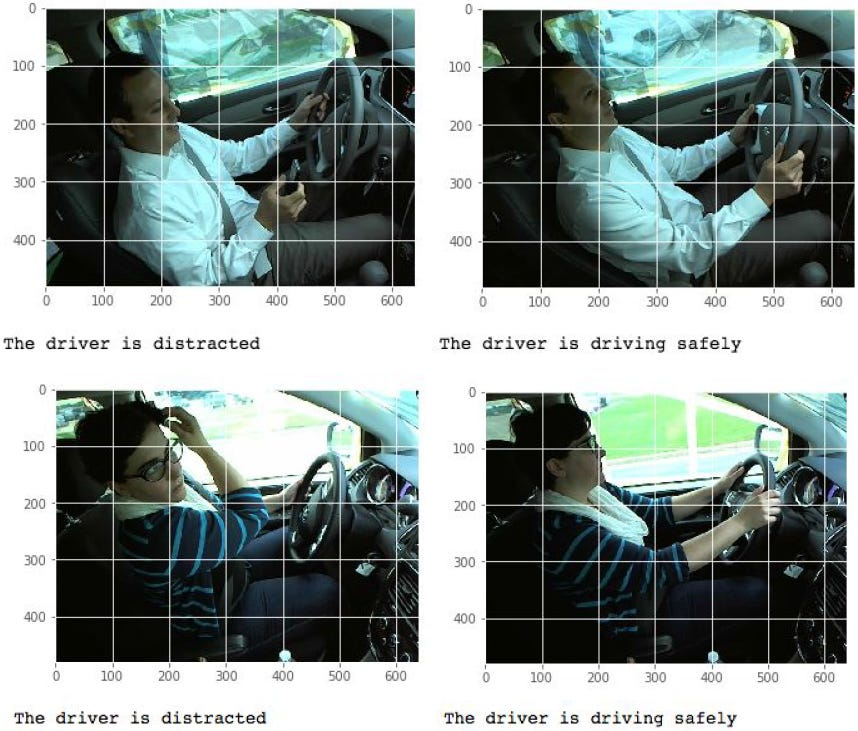Figure 5. Classified images by the final solution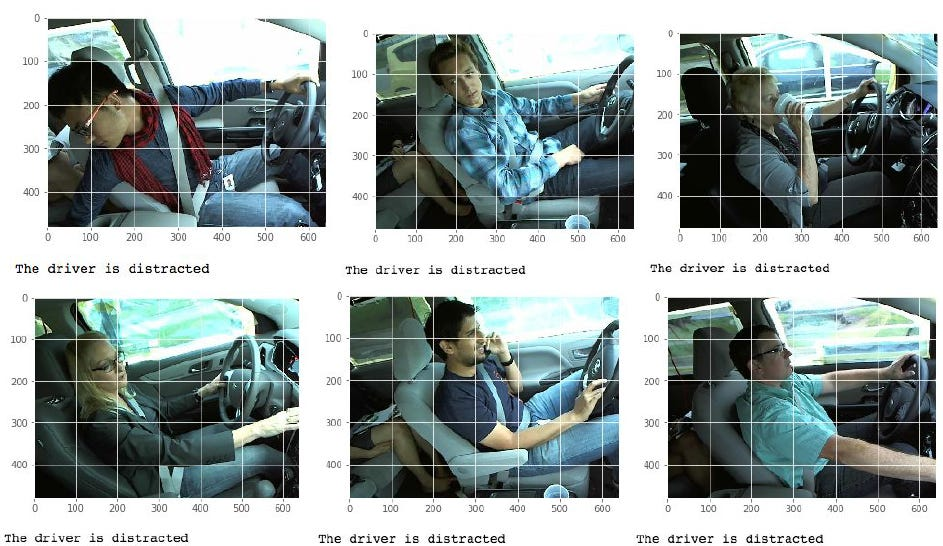Figure 6. Distracting activities identified by the final solution.

#### V.II. Reflection

The raw data was obtained from Kaggle, then they were divided into training, validation and testing sets. These sets were explored, counted, their labels’ distributions were calculated and some examples were displayed to get a general understanding of the data. The data was converted into 4D arrays and scaled. A simple benchmark model was created to get a sense of the problem’s complexity then an initial solution using a pre-trained model as a feature extractor was tried, which was refined by adding an extra fully connected layer before classification. Finally, a solution using a fine-tuned pre-trained model was implemented.

One interesting aspect was that further training the initial solution resulted in a lot worse result not just on the validation and testing sets but also on the training set. The final solution’s results were disappointing as they were not significantly better than the simple benchmark model’s results that was only trained for 4 epochs whereas the final solution model was trained for over 27 hours.

#### V.III. Improvement

The project can be improved by test other pre-trained models such as ResNet50 or InceptionResNetV2. Additionally, testing other optimizers with smaller learning rate and higher momentum. Most importantly is training for much more epochs and probably fine-tune less deeper layers. One interesting experiment would be to initialize the weights of the classifier layer of the final solution’s model with the classifier’s weights from the initial solution to reduce the training time.

### References

1. + Nhtsa.gov. (2018). [online] Available at: https://www.nhtsa.gov/sites/nhtsa.dot.gov/files/documents/812_381_distracteddriving2015.pdf [Accessed 6 Jan. 2019].
2. Cs231n.github.io. (2018). CS231n Convolutional Neural Networks for Visual Recognition. [online] Available at: http://cs231n.github.io/convolutional-networks/ [Accessed 6 Jan. 2019].
3. Cs231n.github.io. (2018). CS231n Convolutional Neural Networks for Visual Recognition. [online] Available at: http://cs231n.github.io/transfer-learning/ [Accessed 6 Jan. 2019].
4. Blog.keras.io. (2018). Building powerful image classification models using very little data. [online] Available at: https://blog.keras.io/building-powerful-image-classification-models-using-very-little-data.html [Accessed 7 Jan. 2019].
5. Gist. (2018). Fine-tuning a Keras model. Updated to the Keras 2.0 API.. [online] Available at: https://gist.github.com/fchollet/7eb39b44eb9e16e59632d25fb3119975 [Accessed 7 Jan. 2019].